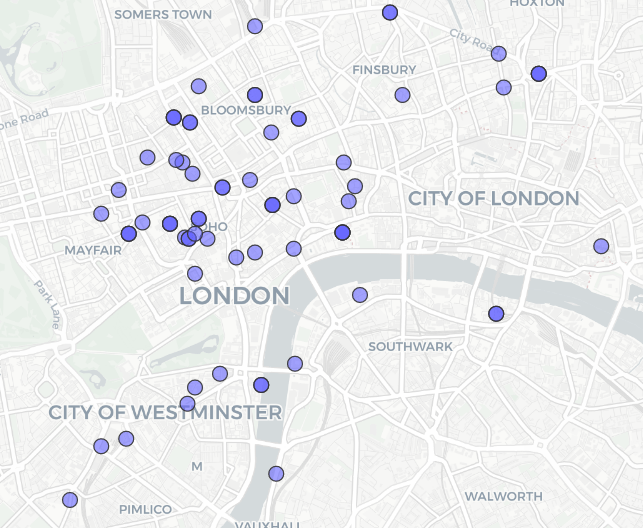# A Decade of Drinking Beer on Untappd

The answers came in swiftly - Untappd was the app to use. So, a few minutes later:

In the last decade, how much beer and cider have I drunk?

I've written before about how to extract your data from Untappd using their API.

(A few notes. I don't check in to every drink - I only tend to do so if it's a new beer. Some of these are only tasters of a beer - not a full pint. This is mostly an exercise in playing with R. Visit DrinkAware if you'd like to help manage your alcohol consumption.)

## Quick Stats

• 985 check ins.
• 801 unique drinks
• 3.68 average rating
• 4.93 average ABV

## Graphs

Is there a correlation between how strong a drink is, and how much I like it?

``````library(jsonlite)
beer_data <- read_json("untappd_data.json", simplifyVector = TRUE)

abv <- beer_data\$beer\$beer_abv
scr <- beer_data\$rating_score

plot(abv, scr, main="ABV vs Score", xlab="ABV", ylab="Score")
abline(lm(scr~abv), col="red") # regression line (y~x)
``````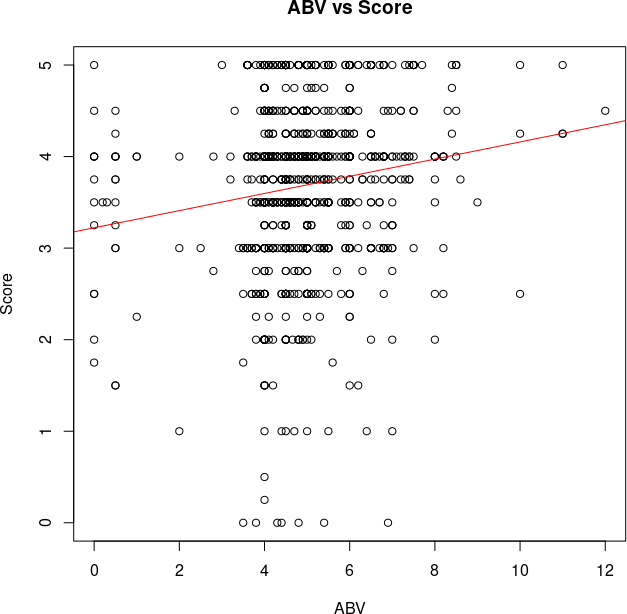Hmmm... There's some week positive correlation there. But it's a bit muddled. Let's turn that into a hexmap:

``````library(hexbin)
bin<-hexbin(abv, scr, xbins=10, xlab="ABV", ylab="Score")
plot(bin, main="Hexagonal Binning")
``````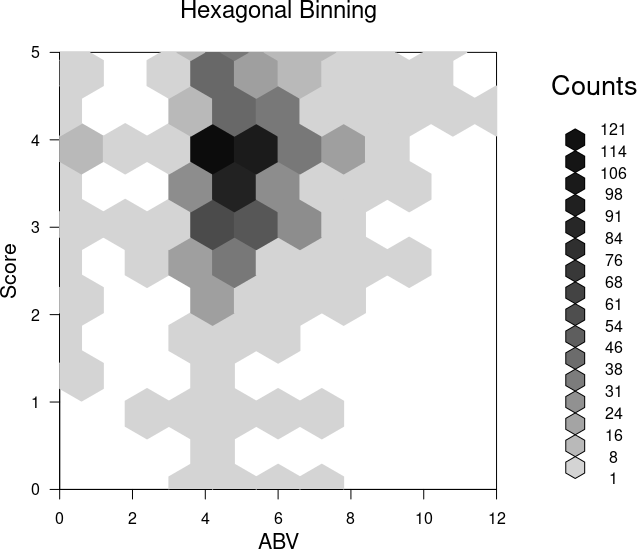Aha! A bit easier to see. Most of the beers I drink are in the 4-5% ABV. And there is some correlation. But, mostly, I just like beer and cider. Hmmm... Which do I prefer?

Let's take a look at Cider first:

``````library(data.table)
beer_data <- read_json("untappd_data.json", simplifyVector = TRUE)
cider <- beer_data[grepl("Cider", beer_data\$beer\$beer_name), ]
cabv <- cider\$beer\$beer_abv
cscr <- cider\$rating_score
``````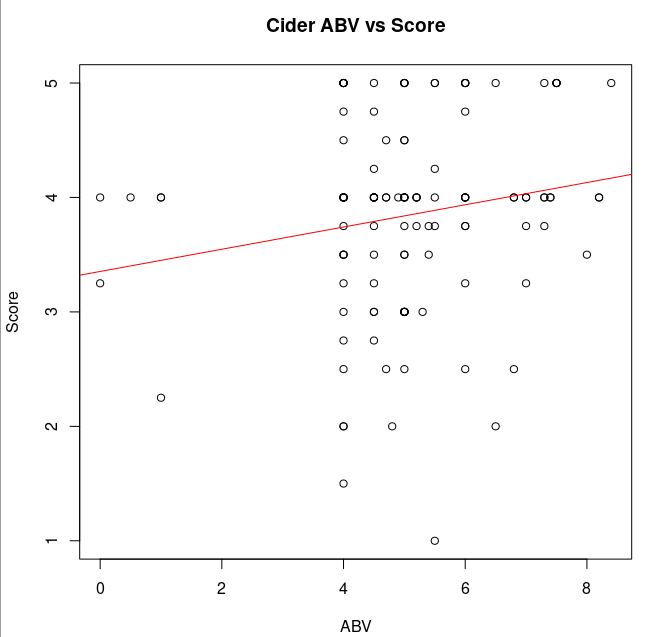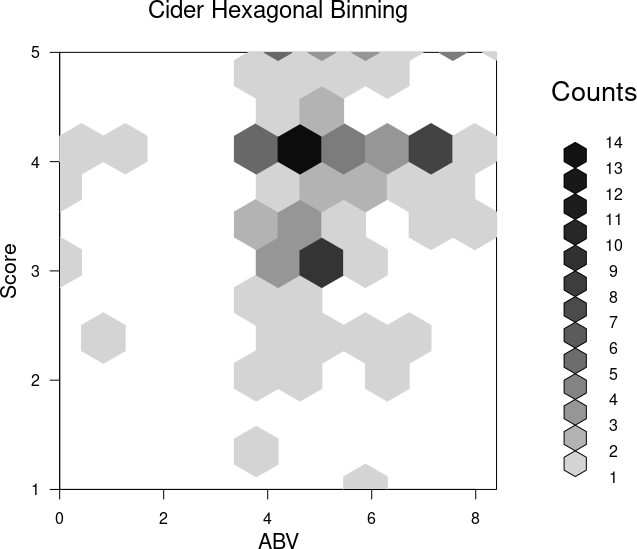How much do I like Cider vs Beer?
Just beer (OK, also includes Mead and a few other not Ciders)

``````justbeer <- beer_data[!grepl("Cider", beer_data\$beer\$beer_name), ]
boxplot(cscr, ylab="Score", main="Cider Scores", ylim=c(0,5))
``````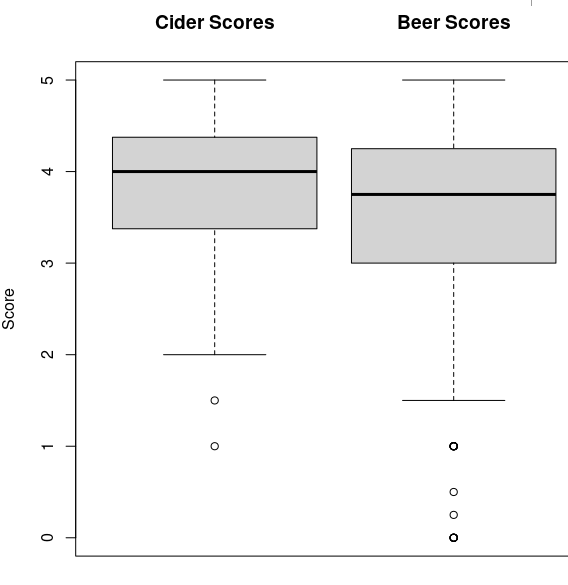I like Cider a bit more than beer. Yup!

Let's plot that data on a map! It's a bit more complicated because the JSON is nested.

``````library(jsonlite)
beer_data <- read_json("untappd_data.json", simplifyVector = TRUE, flatten = TRUE)

venues_list <- beer_data\$venue
venues <- as.data.frame(do.call(rbind, venues_list))
locations_list <- venues\$location
locations <- as.data.frame(do.call(rbind, locations_list))
locations <-subset(locations, venue_state!="Everywhere")
``````

Display them on an interactive map:

``````library(sf)
library(mapview)
locations_sf <- st_as_sf(locations, coords = c("lng", "lat"), crs = 4326)
mapview(locations_sf)
``````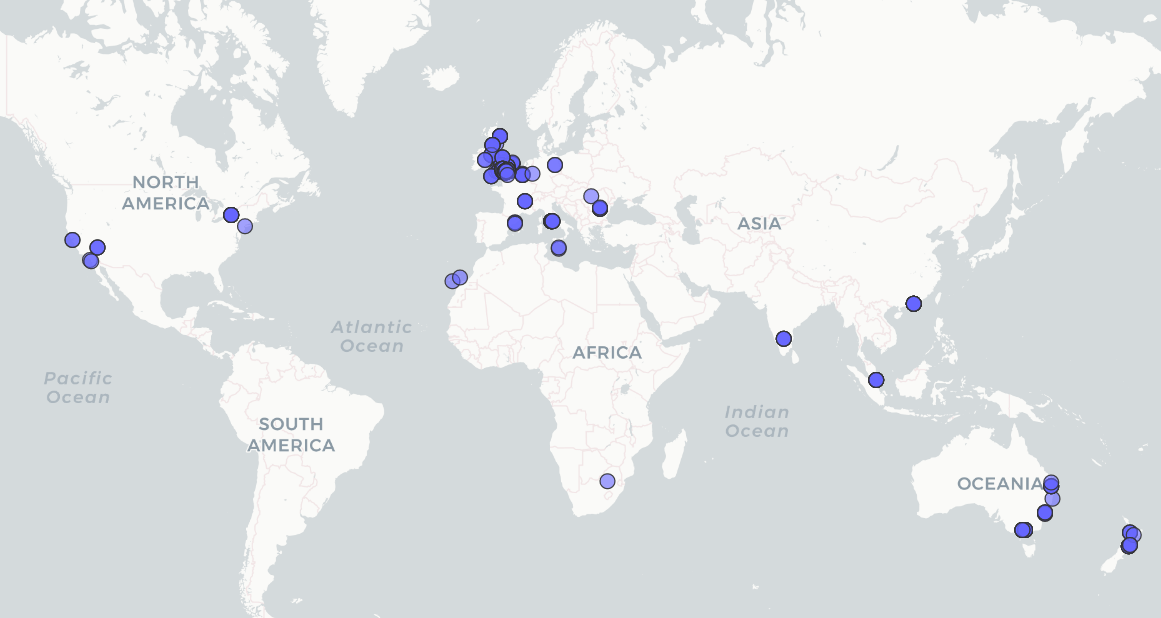Let's zoom in on London: